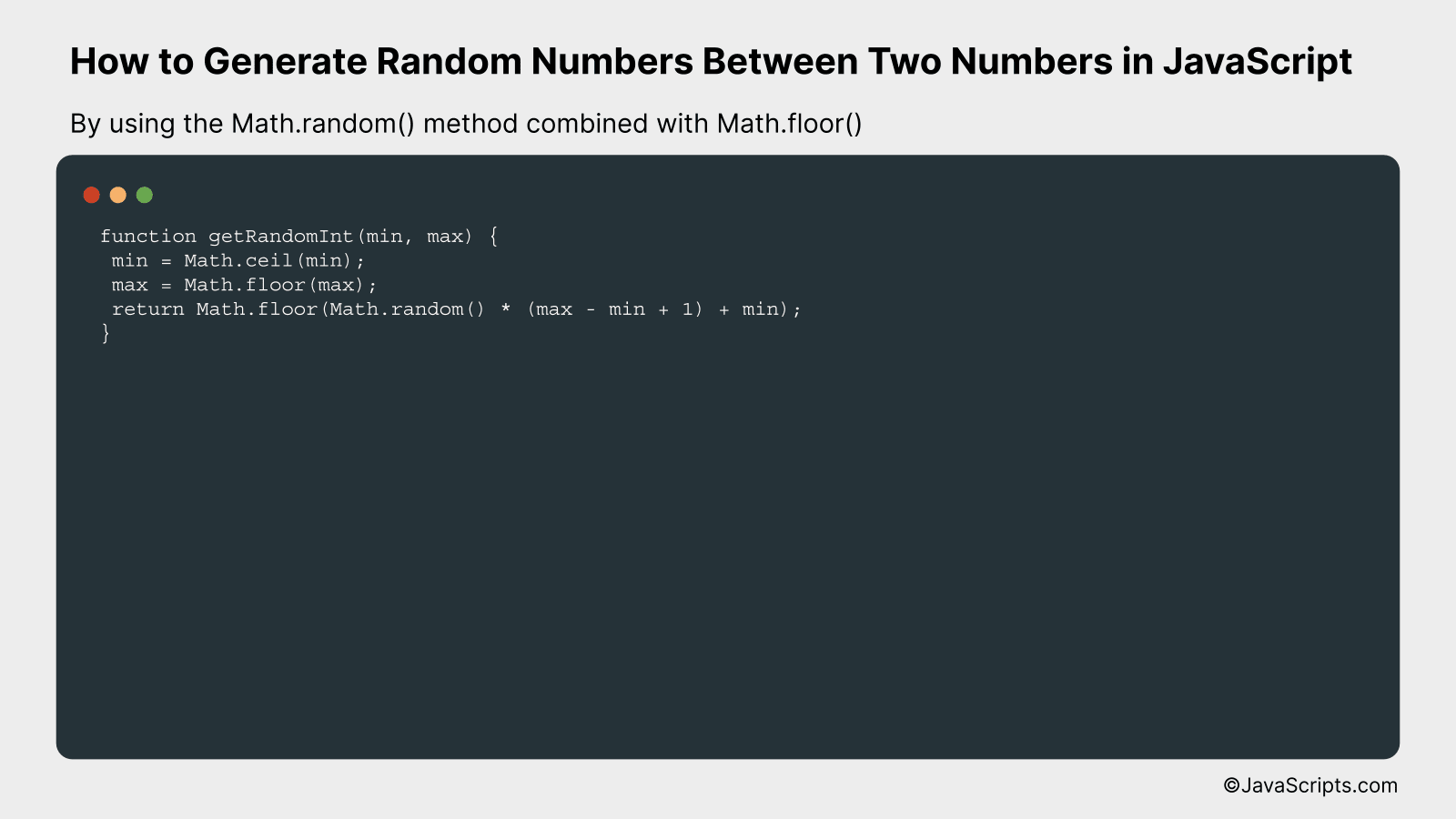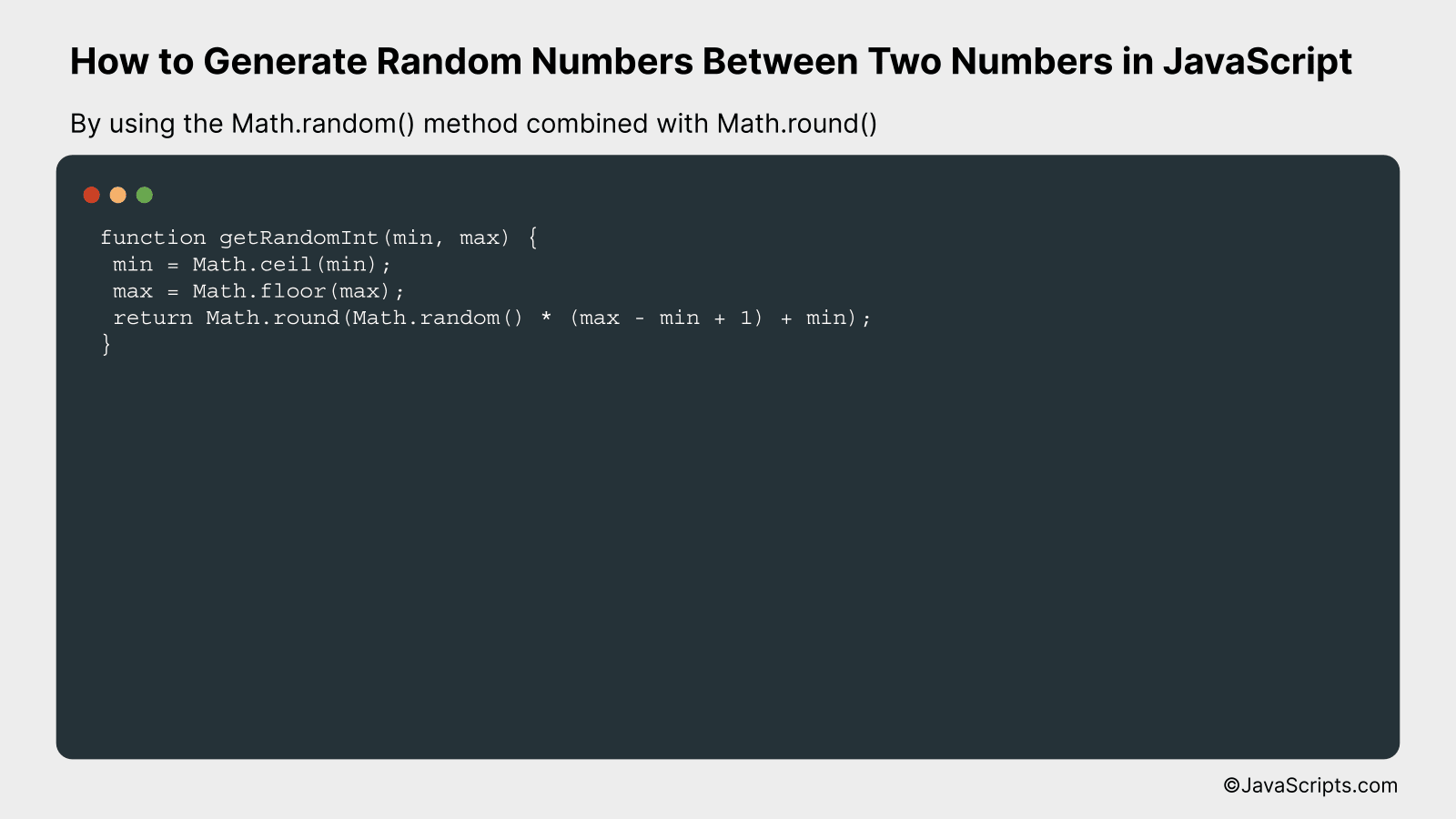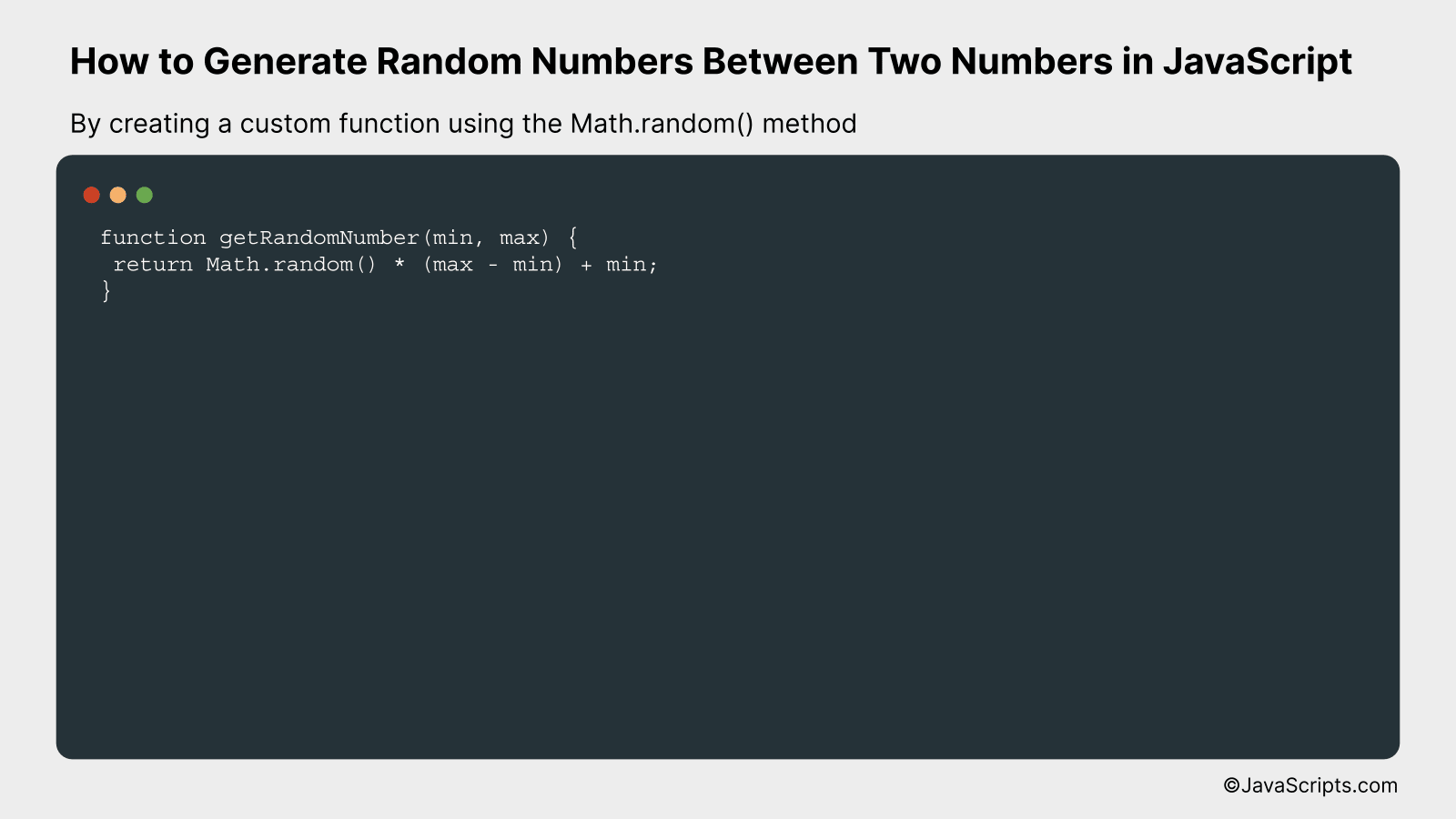# How to Generate Random Numbers Between Two Numbers in JavaScript

Are you dabbling in JavaScript and wondering how to generate random numbers between two given numbers? You’ve come to the right place! It’s an essential skill with a broad range of applications, from developing games to running simulations.

Don’t worry if you’re a beginner. We’ll take a simple, step-by-step approach to understand this concept. Together, we’ll explore the process and you’ll be generating your own random numbers in no time!

In this post, we will be looking at the following 3 ways to generate random numbers between two numbers in JavaScript:

• By using the Math.random() method combined with Math.floor()
• By using the Math.random() method combined with Math.round()
• By creating a custom function using the Math.random() method

Let’s explore each…

## #1 – By using the Math.random() method combined with Math.floor()The Math.random() method generates a random floating point number between 0 (inclusive) and 1 (exclusive), and the Math.floor() method rounds down to the nearest whole number. Combining these two methods with some arithmetic will allow us to generate a random integer between two specific numbers. For example, to generate a random integer between 1 and 10, we’ll adjust the range and rounding of the random number.

``````
function getRandomInt(min, max) {
min = Math.ceil(min);
max = Math.floor(max);
return Math.floor(Math.random() * (max - min + 1) + min);
}
``````

### How it works

We’re defining a function that takes two parameters, min and max. This function generates a random integer within the range [min, max] by first rounding min up and max down to ensure they are integers, then scaling the output of Math.random() to the range of max – min + 1, and finally adding the min value.

• Math.ceil(min): This ensures that min is rounded up to the nearest whole number.
• Math.floor(max): This ensures that max is rounded down to the nearest whole number.
• Math.random(): This method generates a random floating point number between 0 (inclusive) and 1 (exclusive).
• Math.random() * (max – min + 1): This scales the output of Math.random() to the desired range size. The “+1” is to ensure that the max value is included in the possible output values.
• Math.floor(Math.random() * (max – min + 1) + min): This rounds down the result to an integer and shifts the range to start from min value.

## #2 – By using the Math.random() method combined with Math.round()This JavaScript code generates a random integer between two given numbers (inclusive) using Math.random() and Math.round() methods. For example, if we need a number between 1 and 10, this code will provide a random integer from 1 to 10.

``````
function getRandomInt(min, max) {
min = Math.ceil(min);
max = Math.floor(max);
return Math.round(Math.random() * (max - min + 1) + min);
}
``````

### How it works

The getRandomInt function takes in two parameters: ‘min’ and ‘max’. It generates a random number between these two values (inclusive) by using the Math.random() method in combination with the Math.round() method.

• First, the ‘min’ and ‘max’ values are prepared. The Math.ceil() method rounds the ‘min’ value up to the nearest integer, and the Math.floor() method rounds the ‘max’ value down to the nearest integer. This ensures that the random number generated is within the bounds of ‘min’ and ‘max’ even after rounding.
• Then, Math.random() generates a random decimal number between 0 (inclusive) and 1 (exclusive).
• This random decimal is multiplied by the range (max – min + 1) to spread the possible result values across the required range.
• To this product, we add the ‘min’ value to shift the results up to the desired range.
• Finally, Math.round() is used to round the resulting number to the nearest integer, which is then returned by the function.

## #3 – By creating a custom function using the Math.random() methodWe are going to create a JavaScript function that generates a random number between two given numbers. This is done by utilizing the built-in Math.random() method, which generates a random floating-point number between 0 and 1. After getting this random number, we scale it up to our desired range, and then shift it to start from our lower bound. For example, if we want a random number between 5 and 15, we’ll generate a random number, multiply it by 10 (the size of our range: 15-5), and then add 5 (our lower bound) to shift the range.

``````
function getRandomNumber(min, max) {
return Math.random() * (max - min) + min;
}
```
```

### How it works

The provided function takes two arguments, ‘min’ and ‘max’, which are the boundaries of the range within which we want to generate a random number. It uses Math.random() to generate a random floating-point number between 0 and 1, multiplies it by the size of the desired range (max – min), then adds the ‘min’ value to shift the range. This ensures the final result is a random number falling in the correct range.

• Math.random() generates a random floating-point number between 0 (inclusive) and 1 (exclusive).
• We then multiply this random number by the size of the desired range (max – min). This scales up the random number to the correct range size. However, this result would still start from 0.
• To shift this range to start from our desired ‘min’ value, we add ‘min’ to the result. This ensures that our final random number falls between ‘min’ and ‘max’.

Related:

In conclusion, generating random numbers between two specific numbers in JavaScript is a straightforward task. By using the Math.random() function along with a bit of calculation, we can easily produce random numbers within a specified range.

Remember, practice makes perfect. So, keep coding and exploring different functions in JavaScript. With consistent effort, you’ll find JavaScript to be a powerful tool in your programming arsenal.